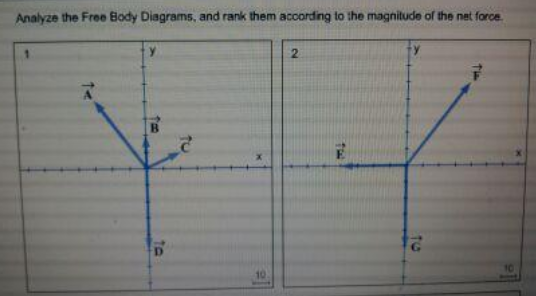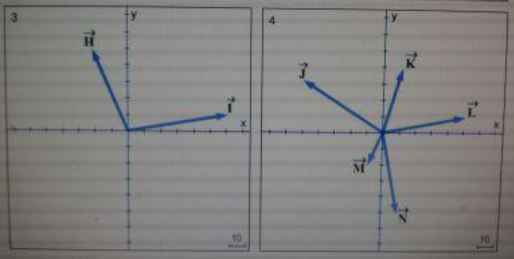# Problem: There are four body diagrams, and rank them according to the magnitude of the net force. From smallest to largest.

###### FREE Expert Solution

1.

A = -3 i + 4j

B = 2j

C = 2i + j

D = -5j

A + B + C + D = (-3i + 2i) + (4j + j - 5j) = -i

|A + B + C + D| = 1

The magnitude of the net force on 1 is 1

89% (4 ratings)###### Problem Details

There are four body diagrams, and rank them according to the magnitude of the net force. From smallest to largest.Frequently Asked Questions

What scientific concept do you need to know in order to solve this problem?

Our tutors have indicated that to solve this problem you will need to apply the Forces in 2D concept. You can view video lessons to learn Forces in 2D. Or if you need more Forces in 2D practice, you can also practice Forces in 2D practice problems.